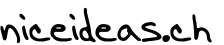#Technological Thoughts by Jerome Kehrli

## Introduction to mathematical optimization

by Jerome Kehrli

Posted on Monday Mar 26, 2012 at 12:30AM in General

During my MSc studies, I followed an extended set of very interesting lectures related to Mathematical Optimization using basic mathematic concepts and simple algorithms such as the Newton (and/or Newton-based) methods or the simplex algorithm (and/or simplex based such as branch-and-bound, branch-and-cut, etc.).

Quoting Wikipedia:

"In the simplest case, an optimization problem consists of maximizing or minimizing a real function by systematically choosing input values from within an allowed set and computing the value of the function. The generalization of optimization theory and techniques to other formulations comprises a large area of applied mathematics.
More generally, optimization includes finding best available values of some objective function given a defined domain, including a variety of different types of objective functions and different types of domains.
"

I have these days a (very) little more than usual free time and I've compiled a resume of these lectures from my various notes and individual chapters resumes. So I decided to put this document online as it might help some of the future MSc students following any lecture related to Mathematical Optimization by providing them with an introduction to the field.

The resume is available here : resume_optim.pdf.

No one has commented yet.